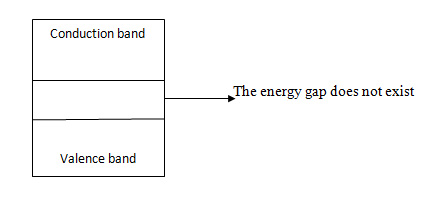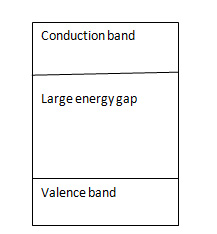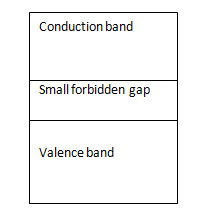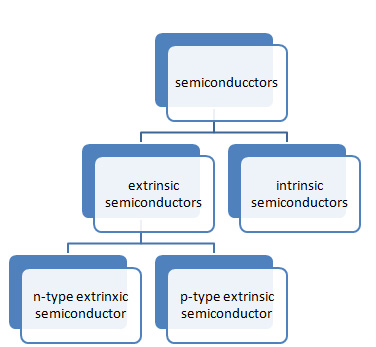Semiconductors PPT and PDF Report Free: The semiconductors have the conductivity which is between the conductors and the insulators. A unique feature of semiconductors is that they are bipolar in nature and in them, the current is transported by the electrons and holes. The free electrons in the outermost orbit are known as valence electrons and these valence electrons determine the properties of the material that whether it is a conductor or semiconductor or insulator.

## Semiconductors PPT and PDF Report Free

The valence electrons in different materials and their properties are explained below:

• The conductors have less than four valence electrons and examples of them are sodium and magnesium.
• The insulators have more than four valence electrons and examples of them are nitrogen, neon, and sulfur.
• The semiconductors have four valence electrons and examples of these materials are silicon and germanium.

Also See: OLED Technology Seminar PPT

### Energy Band Theory:

The energy bands present in the solids are as below:

• Valence band
• Conduction band
• Forbidden gap
1. Valence band: The energy band formed due to a merging of energy levels associated with the valence electrons in the last shell is called a valence band.
2. Conduction band: The energy band formed due to the merging of energy levels associated with the free electrons is called as the conduction band.
3. Forbidden gap: The energy gap which divides the conduction band and valence band is known as forbidden band or forbidden gap. The energy associated with forbidden band is called as energy gap and is denoted by and is measured in electron volt (eV). Therefore, 1ev = 1.62* 10^-19. In the conductors, the forbidden gap overlap and the energy gap will be large in the insulators. In the semiconductors, the energy gap will be very small. The diagrammatical representation of these energy gaps are explained below:Fig1: Energy Band Theory of ConductorsFig2: Energy Band Theory of ConductorsFig3: Energy Band Theory of Insulators

Also See: Augmented Reality Seminar and PPT

### Classification of Semiconductors:

The types of semiconductors are explained below:Fig4: Types of Semiconductors

1. Intrinsic semiconductors: The intrinsic semiconductors are pure semiconductors. At room temperature, some of the valence electrons may acquire sufficient energy to enter the conduction band to acquire sufficient energy to enter the conduction band to form free electrons. Under the influence of electric field, these electrons constitute an electric current. A missing electron in the valence band leaves a vacant space called the hole. The hole also contributes to the electric current and the electron-hole pairs will be formed. When an electric field is applied across an intrinsic semiconductor, the current conduction takes place by two processes namely the free electrons and holes. The energy required to break the covalent bond for germanium is 0.72ev and for silicon, it is 1.1ev at room temperature. The free electrons and holes get generated in pairs. The generation of the electron-hole pair due to the thermal energy is called as the thermally generated electron-hole pair. The number of free electrons and holes are always equal in intrinsic semiconductors. The electrons are negatively charged particles and the holes are positively charged particles.
2. Extrinsic semiconductors: The current conduction capability should be increased by a doping process. Doping is nothing but the adding of a small amount of impurity to the intrinsic semiconductors. So, after the doping, the material becomes an impure or extrinsic semiconductor. Depending upon the type of doping, the extrinsic semiconductor are two types and they are:
• P-type extrinsic semiconductor and
• N-type extrinsic semiconductor

Also See: Solar Mobile Charger Seminar ppt

When pentavalent atoms are added to an intrinsic semiconductor then it is called as donor doping as each impurity atom donates one free electron to an intrinsic material and such impurity is called donor impurity. This makes an extrinsic semiconductor with a large number of free electrons called “n-type extrinsic semiconductor.” Examples of such materials are arsenic, bismuth, and phosphorus.

When trivalent atoms are added to intrinsic semiconductors then it creates more holes and will be ready to accept an electron. Hence this type of doping is called as acceptor doping and this result in the “p-type extrinsic semiconductor.” Examples of such materials are gallium, boron, and indium.

• N-type extrinsic semiconductor: If a small amount of pentavalent impurity is added to a semiconductor then it is called as the n-type extrinsic semiconductor. The pentavalent impurity has five valence electrons. When the voltage is applied to the n-type semiconductor, the free electrons which are readily available due to the included impurity move in a direction of positive terminal of the applied voltage. This constitutes a current and the conduction is by free electrons. In this, the holes are less in number and the current due to electrons is dominant over the hole current.
• P-type extrinsic semiconductor: A few trivalent impurities like aluminum or boron and gallium is added to a pure semiconductor to get a p-type extrinsic semiconductor. The trivalent impurities are called as acceptors because it accepts free electrons in the place of holes. The free electrons are also present in the conduction band but are very less in number and in this, holes are the majority carriers and the electrons are the minority carriers.

Also See: Electrophoresis Ppt Seminar

### Content of the Seminar and PDF Report for Semiconductors

• Introduction
• Types OF Semiconductors
• Intrinsic
• Extrinsic
• N-Type Material
• P-Type material
Classification of materials
• Band diagram
• Current flow in semiconductor
• References

Here we are giving you Semiconductors Seminar and PPT with PDF report. All you need to do is just click on the download link and get it.

ECE SeminarsSemiconductors PPT and PDF Report Free: The semiconductors have the conductivity which is between the conductors and the insulators. A unique feature of semiconductors is that they are bipolar in nature and in them, the current is transported by the electrons and holes. The free electrons in the outermost...